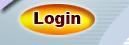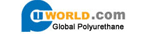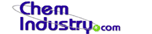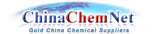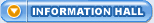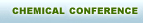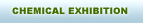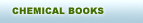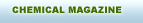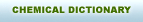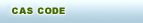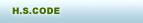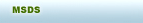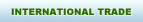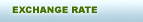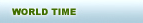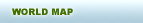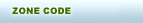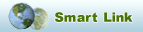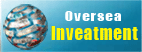HOME | SITE MAP

 Site: Index >> Information >> Chemical Dictionary

Sourcing by alphabetical listing:
 A B C D E F G H I J K L M N O P Q R S T U V W X Y Z
Chemistry Dictionary Terminology "A"
 Chemistry Dictionary Terminology  "M" Magnetic Quantum Number (mc) Quantum mechanical solution to a wave equation that designates the particular orbital within a given set (s, p, d, f ) in which a electron resides. Manometer A two-armed barometer. Mass A measure of the amount of matter in an object. Mass is usually measured in grams or kilograms. Mass Action Expression For a reversible reaction, aA + bB cC + dD the product of the concentrations of the products (species on the right), each raised to the power that corresponds to its coefficient in the balanced chemical equation, divided by the product of the concentrations of reactants (species on the left), each raised to the power that corresponds to its coefficient in the balanced chemical equation. At equilibrium the mass action expression is equal to K; at other times it is Q.[C]c[D]d [A]a[B]b = Q, or at equilibrium K Mass Deficiency The amount of matter that would be converted into energy if an atom were formed from constituent particles. Mass Number The sum of the numbers of protons and neutrons in an atom; an integer. Mass Spectrometer An instrument that measures the charge-to-mass ratio of charged particles. For further information see Mass Spectrometry Matter Anything that has mass and occupies space. Mechanism The sequence of steps by which reactants are converted into products. Melting Point The temperature at which liquid and solid coexist in equilibrium; also the freezing point. Meniscus The shape assumed by the surface of a liquid in a cylindrical container. Metal An element below and to the left of the stepwise division (metalloids) in the upper right corner of the periodic table; about 80% of the known elements are metals. Metallic Bonding Bonding within metals due to the electrical attraction of positively charges metal ions for mobile electrons that belong to the crystal as a whole. Metallic Conduction Conduction of electrical current through a metal or along a metallic surface. Metalloids Elements with properties intermediate between metals and nonmetals: B, Al, Si, Ge, As, Sb, Te, Po, and At. Metallurgy Refers to the overall processes by which metals are extracted from ores. Metathesis Reactions Reactions in which two compounds react to form two new compounds, with no changes in oxidation number. Reactions in which the ions of two compounds exchange partners. Method of Initial Rates Method of determining the rate-law expression by carrying out a reaction with different initial concentrations and analyzing the resultant changes in initial rates. Miscibility The ability of one liquid to mix with (dissolve in) another liquid. Mixture A sample of matter composed of two or more substances, each of which retains its identity and properties. Moderator A substance such as hydrogen, deuterium, oxygen or paraffin capable of slowing fast nuetrons upon collision. Molality Concentration expressed as number of moles of solute per kilogram of solvent. Molarity Number of moles of solute per litre of solution. Molar Solubility Number of moles of a solute that dissolve to produce a litre of saturated solution. Molecular Equation Equation for a chemical reaction in which all formulas are written as if all substances existed as molecules; only complete formulas are used. Molecular Formula Formula that indicates the actual number of atoms present in a molecule of a molecular substance. Molecular Geometry The arrangement of atoms (not lone pairs of electrons) around a central atom of a molecule or polyatomic ion. Molecular Orbital An orbit resulting from overlap and mixing of atomic orbitals on different atoms. An MO belongs to the molecule as a whole. Molecular Orbital Theory A theory of chemical bonding based upon the postulated existence of molecular orbitals. Molecular Weight The mass of one molecule of a nonionic substance in atomic mass units. Molecule The smallest particle of an element or compound capable of a stable, independent existence. Mole Fraction The number of moles of a component of a mixture divided by the total number of moles in the mixture. Monoprotic Acid Acid that can form only one hydronium ion per molecule; may be strong or weak. Acid that contains one ionizable hydrogen atom per formula unit. Mother Nuclide Nuclide that undergoes nuclear decay.

 Chemical:     (Powered by Chemindustry.com) Enter a chemical name, CAS Number, or molecular formula. Use * for partial names (i.e. chloro*)Welcome to the Hichem.com Chemical Name Synonyms finder. Enter any of the following options in the search box: a complete chemical name   e.g. Chlorosulfuric acid. a partial name   e.g. 1 chloro*, e.g. 2 *chloride. a CAS number   e.g. 50-00-0. a molecular formula   e.g. C6H6.
Popular Chemicals
A B C D E F G H I J K L M N O P Q R S T U V W X Y Z

# General Chemistry Glossary

Browse alphabetically: A B C D E F G H I J K L M N O P Q R S T U V W X Y Z